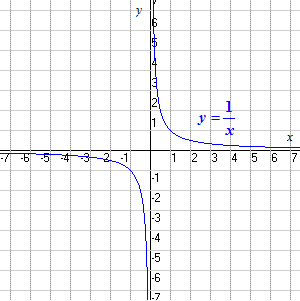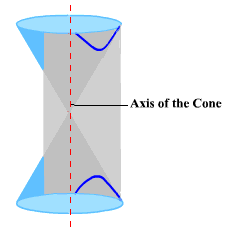# Hyperbolas

The "basic" hyperbola is the function

$y=\frac{1}{x}$

This function has a graph which consists of two disjoint parts. Note that $0$ is not in the domain . Note also that the function approaches $0$ asymptotically as $x$ grows infinitely large (or infinitely negative), and that it approaches infinity as $x$ approaches $0$ from the positive side, negative infinity as $x$ approaches $0$ from the negative side.The center of the hyperbola is the point of rotational symmetry. In the above example, the center is $\left(0,0\right)$ .

The graph of the rational function

$y=\frac{a}{x-h}+k$

is a hyperbola whose center is $\left(h,k\right)$ . The constant $a$ controls the "steepness" with which the graph approaches the asymptotes .

A hyperbola can also be defined as a conic section obtained by the intersection of a double cone with a plane that is intersects both pieces of the cone without intersecting the axis.